Next: Variables Up: Plot Specification Previous: Output Noise Spectrum   Contents

### Sensitivities

For the determination of the sensitivity of the transfer function of the filter for component variations without Monte Carlo analysis, three functions are provided. These can be specified by the keywords relsens, abssens and sens. They stand for the following quantities.

 relsens2 =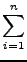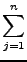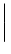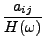⋅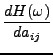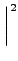+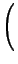⋅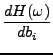+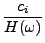⋅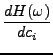(9)

 sens2 =aij⋅+bi⋅+ci⋅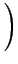(10)

 abssens2 =++(11)

The state-space parameters of the filter (the aij , bi and ci ) are in practice realized by resistances or transconductances in combination with capacitors. Therefore the deviations of the components of the filter are reflected in its state space parameters. The particular function that is used to determine the deviations in the transfer function of the filter depends on the assumptions on the nature of the component deviations.

relsens is the ratio of the total relative standard deviation of the transfer function of the filter to the relative standard deviation of the aij , the bi and the ci . So relsens applies to a situation where the components suffer from the same relative deviation.

This is also the assumption underlying the use of sens. The difference is that the absolute deviation of the transfer function is computed (which should still be multiplied by the relative deviation of the component values).

If the components suffer from the same absolute deviation abssens should be used.

The functions relsens2, sens2, and abssens2 are similar to relsens, sens, and abssens. The difference is that the former do not take the contributions due to the bi and the ci into account. This might be useful if (like in the example of section 6.1) there is only one bi and only one ci unequal to zero. In that case, variations in these branches will not alter the `shape' of the filter, but only its gain.Next: Variables Up: Plot Specification Previous: Output Noise Spectrum   Contents
2009-06-03